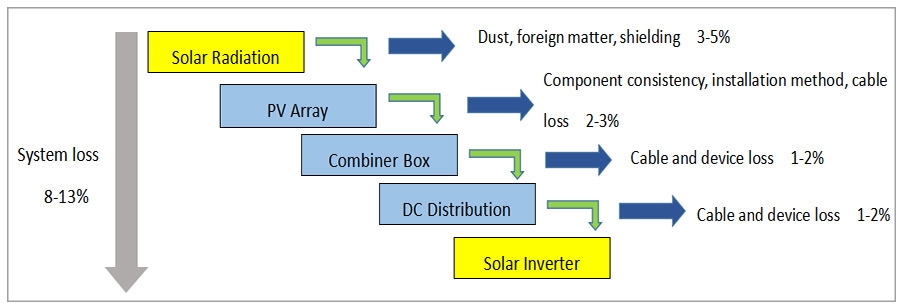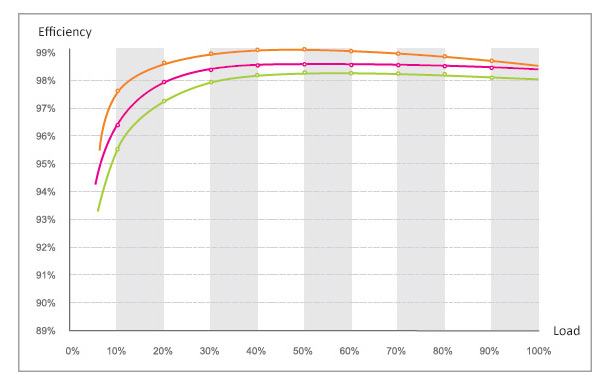# 5 Factors Affect PV Module and Inverter Capacity Ratio

The PV module capacity and solar inverter capacity ratio are commonly referred to as capacity ratio. Reasonable capacity ratio design needs to be considered comprehensively in the light of the specific project. The main influencing factors include irradiance, system loss, inverter efficiency, inverter life, inverter voltage range, assembly installation angle and so on. Because the inverter accounts for only about 5% of the system cost, the number or power of the inverter is reduced by the overmatching of the components in the distributed photovoltaic power station system, and the investment income is very small, which also bring other problems, the specific analysis is as follows.

Different irradiance in the different regions

According to the standard of wind energy and solar energy evaluation center of National Meteorological Administration, the solar energy resources in China are divided into four categories, and the irradiance of different regions is quite different. Even the annual radiation levels vary greatly from place to place in the same resource area. For example, the total annual radiation of the Gaer Tibet and Golmud, Qinghai, the resource area of Class I, Gaer is 7998 MJ/m2, which is 17% higher than Golmud's 6815 MJ/m2. It means that under the same system configuration, that is, the same capacity ratio, the power generation in the Gaer region is 17% higher than that in Golmud. In order to achieve the same power generation, it can be achieved by changing the ratio of capacity to distribution.

System loss

There is a loss in every link of energy from solar radiation to photovoltaic modules, through DC cables, confluence boxes, DC distribution to solar inverters in photovoltaic system. As shown in the figure, the DC side loss is usually about 7-11%, the inverter loss is about 1-2%, and the total loss is about 8-13%. (the system loss mentioned here does not include the transformer and line loss behind the inverter).

That is to say, under the condition that the module capacity is equal to the solar inverter capacity, due to the objective existence of various losses, the actual maximum output capacity of the inverter is only about 90% of the rated capacity of the inverter, even when the light is the best, the inverter does not work at full load.Solar inverter efficiency

The efficiency of the solar inverter is not constant, there are power switching device losses and magnetic losses. The efficiency is relatively low at low power. When the power is 40% to 60%, the efficiency is the highest, and when the efficiency is more than 60%, the efficiency decreases gradually. Therefore, the total power of photovoltaic power should be controlled between 40% and 60% of inverter power to obtain the best efficiency.Solar inverter life

Photovoltaic solar inverter is an electronic product, its reliability is closely related to the operating temperature of the inverter, of which, the temperature of components, such as capacitance, fan and relay can be increased by 10℃, and the failure rate can be increased by more than 50%. and the operating temperature is related to power. According to statistics, the long-term working power of the inverter is 80-100%, which is about 20% lower than that of the power of 40-60%, and the life of the inverter is about 20% lower than the inverter.

Optimal operating voltage range of Inverter

The working voltage is about the rated working voltage of the solar inverter, and the efficiency is the highest. The single-phase 220V inverter and the inverter input rated voltage are 360V, the three-phase 380V inverter and the inverter input rated voltage re 650V. Such as 3000 watt solar inverter, equipped with 260W module, 30.5V operating voltage, equipped with 12*366V operating voltages, the total power is 3.12kW is the best. The 30kW solar inverter is equipped with 260W module, connected with 126modules, 21 strands each, the voltage is 640.5V, and the total power is 32.76kW.

According to all kinds of factors, the power of the system is between 40 and 60% of the rated power of the solar inverter, the efficiency is the highest and the life is the longest. In order to optimize the performance of the inverter, the components and inverter have different proportions depending on the lighting conditions.

1. The average sunshine time is more than five hours in Class I light area, the power generation time is calculated at 10 hours per day, and the recommended components and solar inverter are configured as 1:1 with an average power of about 50%.
2. The average sunshine time is more than five hours in Class II light area, the power generation time is calculated at 9 hours per day, and the recommended components and inverter are configured as 1.1:1, (4*1.1)/9, with an average power of about 49%.
3. The average sunshine time is more than five hours in Class IIII light area, the power generation time is calculated at 3.5 hours per day, and the recommended components and inverter are configured as 1.2:1, (3.5*1.2)/8.5, with an average power of about 49.4%.
4. The power generation time is calculated at 8 hours a day in Class IIII light area where the average sunshine time is less than 3 hours, and the recommended components and inverter are configured as 1.3:1, (3*1.3)/8, with an average power of about 48.75%.